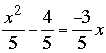SEARCH HOMEMath Central Quandaries & QueriesQuestion from Lorrie, a student: x^2/5 - 4/5 = -3/5xLorrie,

When I look at the equationthe first thing I notice is all the fractions. I would rather not deal with fractions so I would like to eliminate them. Fortunately they all have a denominator of 5 to I can eliminate them by multiplying both sides of the equation by 5. This gives

x2 - 4 = -3 x

Now add 3x to each side to obtain

x2 +3 x - 4 = 0

Can you factor this and solve for x?

PennyMath Central is supported by the University of Regina and The Pacific Institute for the Mathematical Sciences.